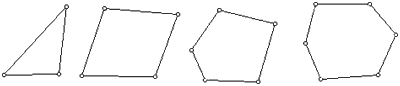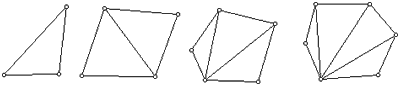# Sum of Angles in a Polygon

What is the sum of the angles of a triangle? of a quadrilateral? of a pentagon? of a hexagon? What is the sum of the angles in convex polygons in terms of the number of sides? Scroll down for a hint.Extension:
Does the relationship for convex polygons also hold true for nonconvex polygons? Explain.Hint: